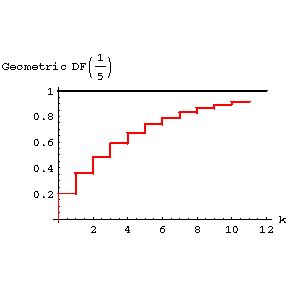# Geometric distribution

2020 Mathematics Subject Classification: Primary: 60E99 [MSN][ZBL]

The distribution of a discrete random variable assuming non-negative integral values $m = 0, 1 \dots$ with probabilities $p _ {m} = pq ^ {m}$, where the distribution parameter $p = 1 - q$ is a number in $( 0, 1)$. The characteristic function is

$$f ( t) = \frac{p}{1 - qe ^ {it} } ,$$

the mathematical expectation is $q/p$; the variance is $q/ p ^ {2}$; the generating function is

$$P ( t) = \frac{p}{1 - qt } .$$Figure: g044230a

A geometric distribution of probability $p _ {m}$.Figure: g044230b

The distribution function $( p = 0.2)$.

The random variable equal to the number of independent trials prior to the first successful outcome with a probability of success $p$ and a probability of failure $q$ has a geometric distribution. The name originates from the geometric progression which generates such a distribution.

How to Cite This Entry:
Geometric distribution. Encyclopedia of Mathematics. URL: http://encyclopediaofmath.org/index.php?title=Geometric_distribution&oldid=47089
This article was adapted from an original article by V.M. Kalinin (originator), which appeared in Encyclopedia of Mathematics - ISBN 1402006098. See original article